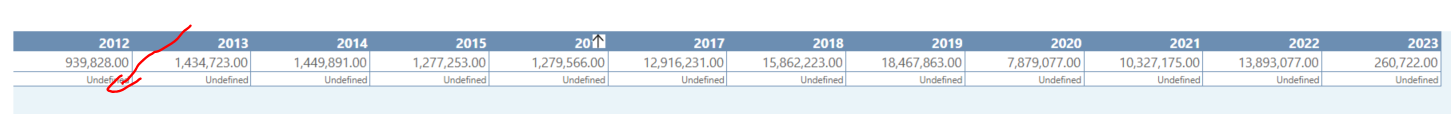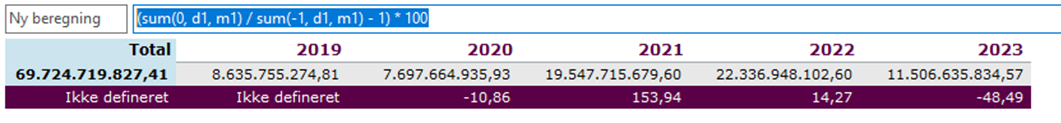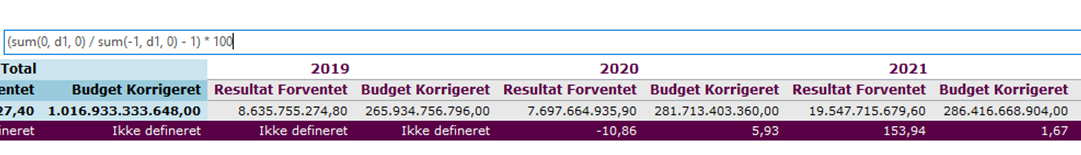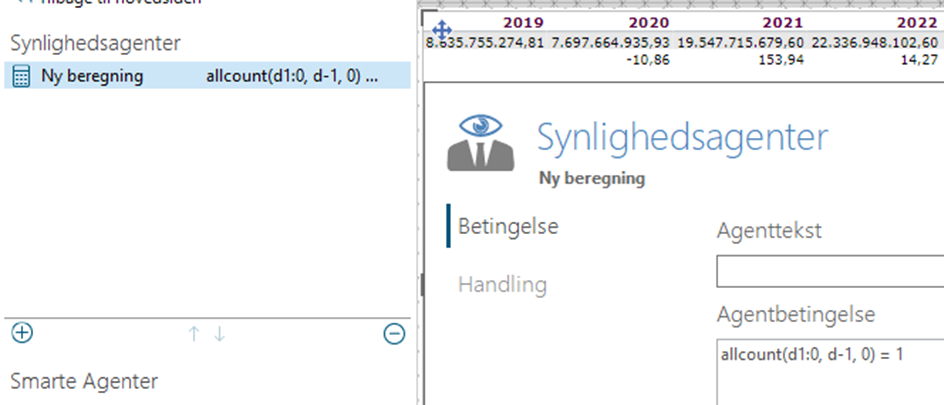# Please can you help meIs it possible to show me how to write the calculation for YoY growth in the line depicted as "undefined"

Thank you

1 comment
• If you just have 1 measure (m1) and 1 row it could be:

(sum(0, d1, m1) / sum(-1, d1, m1) - 1) * 100

or

(sum(0, d-1, m1) / sum(-1, d-1, m1) - 1) * 1000 and -1 refers to the column where 0 is the current column and -1 is the column 1 space to the left.

d1 refers to the row (d1 for the first row or d-1 for the last row - with just 1 row it is the same)

m1 refers to the measure. If you have more than 1 measure, you could use (sum(0, d1, 0) / sum(-1, d1, 0) - 1) * 100 insteadAnd if you want to hide the "undefined" in the first column, you could add a visibility agent that hides the value of the first column:

allcount(d1:0,d1,0) = 1